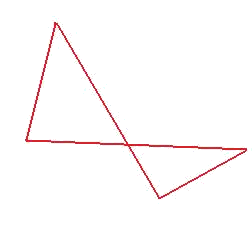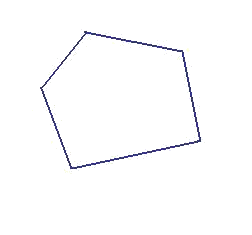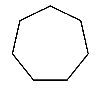# Types of polygons## Simple polygon

A polygon which non adjacent sides do not intersect. An example of a simple polygon is the following image.## Complex polygon

A polygon that is not simple is named a complex polygon. An example of a complex polygon.## Convex polygon

A polygon in which all the interior angles measure less than $$180^\circ$$. All the apexes point towards the exterior of the polygon. We can see an example:## Concave polygon

A polygon that does not satisfy the conditions needed to be classified as convex is named a concave polygon.## Regular polygon

A polygon in which all the sides have the same length and all the interior angles measure the same. All the polygons listed in the table of regular polygons are examples of this type of polygon. Let's see a heptagon:## Irregular polygon

The polygon that is not regular is called irregular polygon.